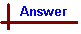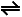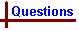Acids and Bases1 - Questions Link to Data Page Your feedback on these self-help problems is appreciated. Click here to send an e-mail. For on-line help on this topic, please see the following Chemcal modules: Acids and Bases (strong acids and bases; conjugate acid/base pairs; pH;self-dissociation of water) Weak Acids and Bases (weak acids; weak bases; Ka and Kb) Calculations with Weak Acids and Bases (calculations involving weak acids and bases and buffers) Acid-base Titrations (titration curves for acid/base titrations; indicators) Shortcut to Questions Q: 1 2 3 4 5 6 7 8 9 10 11 1 Provide the conjugate base for the following acids: (1.) H3O+ (2.) NH4+ (3.) HCl (4.) HCO3- (5.) [Fe(H2O)6]3+ (6.) C6H5OH (7.) HPO42- Provide the conjugate acid for the following bases: (8.) SO42- (9.) O2- (10.) [Al(H2O)5OH]2+ (11.) N(CH3)3 (12.) N3- (13.) H2PO4- (14.) NH32 (1.) Write an equation for the self-ionization of water (2.) Give an algebraic expression for Kw (3.) Define pKw and state its value at 298 K to 2 significant figures.3 For the following solutions, determine: [H+], [OH-], pH, pOH (1.) HBr (1.0 x 10-3 M) (2.) Ba(OH)2 (1.0 x 10-5 M)4 (1.) Write the equation for the equilibrium which is established when propanoic acid (CH3CH2COOH) is added to water. (2.) Write the algebraic expression for KA of propanoic acid. (3.) A water solution of propanoic acid had, at equilibrium, [CH3CH2COOH] = 0.10 M and [H3O+] = 1.2 x 10-3 M. Calculate KA for propanoic acid.5 (1.) Write the equation for the equilibrium which is established when a quantity of ammonia gas is dissolved in water, and (2.) the algebraic expression for KB of ammonia. (3.) A sample of "ammonia water" was 1.0 M in NH3 and 4.2 x 10-3 M in OH-. Calculate KB for ammonia.6 For the weak acid HA: (1.) Write the algebraic expression for KA (2.) Write the algebraic expression for KB of the conjugate base of HA (3.) Show that KB = KW / KA (4.) Calculate KB for CN- given that dissolution of hydrogen cyanide gas in water gave, at equilibrium, [HCN] = 0.010 M and [H3O+] = 2.5 x 10-6 M.7 (1.) Using acid dissociation constant tables, list the following in order of increasing acid strength: H2S, HCN, HCO3-, H2PO4-, HCOOH (2.) Using KA tables for their conjugate acids, list the following in order of increasing base strength: CN-, N2H4, NH3, HS-, H2PO4-, HSO4-8 Write an equation for the self-ionisation of (1.) sulfuric acid (2.) liquid ammonia9 (1.) Write an equation illustrating hydration (2.) Write an equation illustrating hydrolysis10 (1.) Why is Na+ in water solution hydrated to an indefinite rather than a definite degree? (2.) Why is the hydrated Al3+ an acid whereas the hydrated Na+ is not?11 For the acid/base reaction, in water solution, HA + B-A- + HB (1.) Find the expression for the concentration equilibrium constant KC, (2.) Find the condition which must be satisfied for the products to predominate at equilibrium, and (3.) Show that KC = KA(HA) / KA(HB) and that the condition for the products to predominate at equilibrium is given by KA(HA) > KA(HB)Acids and Bases 1 (Answers) 1 A conjugate acid - base pair differ by H+. For example, when H3O+ reacts as an acid and loses a proton, the conjugate base (H2O) is formed: H3O+H2O + H+. Hence H2O is the conjugate base of H3O+ (and, therefore, H3O+ is the conjugate acid of H2O). (1.) H2O (2.) NH3 (3.) Cl- (4.) CO32- (5.) [Fe(H2O)5OH]2+ (6.) C6H5O- (7.) PO43- Conjugate acids are the species formed when a conjugate base accepts a proton. (8.) HSO4- (9.) OH- (10.) [Al(H2O)6]3+ (11.) (CH3)3NH+ (12.) HN3 (13.) H3PO4 (14.) NH4+2 (1.) 2H2OH3O+ + OH- (2.) Kw = [H3O+] [OH-] (3.) pKw = -log Kw = 14.00 at 298 K Note the conversion of significant figures between K and pK - the number of significant figures in K becomes the number of decimal places in the pK value (and vice versa), since pK is a logarithmic scale. So in the example above, 2 significant figures of accuracy in the K data results in 2 decimal places of accuracy in the resultant pK value.3 (1.) HBr is a strong acid, so we assume complete ionisation in solution: HBr + H2O → H3O+ + Br-. Note the use of a single-headed arrow to represent complete reaction to the product side. This implies that the equilibrium concentration of H3O+ equals the initial concentration of HBr, ie [H3O+] = 1.0 x 10-3 M. pH = -log [H3O+] = 3.00 Kw = [H3O+] [OH-]. Therefore [OH-] = Kw / [H3O+] = 1.0 x 10-14 M2 / 1.0 x 10-3 M = 1.0 x 10-11 M. pOH = -log [OH-] = 11.00 (2.) Ba(OH)2 is soluble, releasing the strong base OH- into solution. Ba(OH)2 → Ba2+ + 2OH- [OH-] = 2 x 1.0 x 10-5 M = 2.0 x 10-5 M pOH = -log [OH-] = 4.70 [H3O+] = Kw / [OH-] = 1.0 x 10-14 M2 / 2.0 x 10-5 M = 5.0 x 10-10 M pH = -log [H3O+] = 9.304 (1.) Propanoic acid, CH3CH2COOH, is a weak acid, so the dissociation is a reversible process at equilibrium (unlike strong acids which are considered completely ionised in water). Note that the acidic carboxylic hydrogen is lost: CH3CH2COOH + H2OCH3CH2CO2- + H3O+ (2.) KA = [CH3CH2CO2-] [H3O+] / [CH3CH2COOH] (3.) If [H3O+] = 1.2 x 10-3 M then [CH3CH2CO2-] must also equal 1.2 x 10-3 M (from part (2.)). KA = [CH3CH2CO2-] [H3O+] / [CH3CH2COOH] = (1.2 x 10-3 M)2 / 0.10 M = 1.4 x 10-5 M.5 (1.) Ammonia is a weak base, so the hydrolysis is an equilibrium process. NH3 + H2ONH4+ + OH- (2.) KB = [NH4+] [OH-] / [NH3] (3.) Given [OH-] = 4.2 x 10-3 M, [NH4+] must also equal 4.2 x 10-3 M. ∴ KB = (4.2 x 10-3 M)2 / 1.0 M = 1.8 x 10-5 M.6 (1.) KA = [H3O+] [A-] / [HA] (2.) KB = [OH-] [HA] / [A-] (3.) KW / KA = [H3O+] [OH-] [HA] / [H3O+] [A-] = [OH-] [HA] / [A-] = KB. (4.) HCN + H2OH3O+ + CN- Given [H3O+] = 2.5 x 10-6 M, [CN-] must also equal 2.5 x 10-6 M. KA of HCN = (2.5 x 10-6 M)2 / 0.01 M = 6.25 x 10-10 M. ∴ KB of CN- = KW / KA(HCN) = 1.0 x 10-14 M2 / 6.25 x 10-10 M = 1.6 x 10-5 M.7 (1.) In order of increasing acid strength: HCO3-, HCN, H2PO4-, H2S, HCOOH (2.) In order of increasing base strength: HSO4-, HS-, H2PO4-, N2H4, CN-, NH38 Self ionisation involves the one species acting as both proton donor and proton acceptor: (1.) 2H2SO4HSO4- + H3SO4+ (2.) 2NH3(l)NH2- + NH4+9 (1.) Hydration is the addition of H2O to a molecule to form a new species, for example: · Cu2+ + 4H2O → Cu(H2O)42+ · CaO + H2O → Ca(OH)2 (2.) Hydrolysis is the splitting of water (hence the name hydro-lysis). Note that where hydration involves the addition of one or more H2O molecules, hydrolysis always involves the cleavage of a water O-H bond during reaction, often leading to the formation of H+ or OH-, for example: · Hydrolysis of a hydrated cation: M(H2O)xn+ + H2OH3O+ + M(H2O)x -1(OH)(n -1)+ eg. Fe(H2O)63+ + H2OH3O+ + Fe(H2O)5(OH)2+ · Hydrolysis of a hydrated anion: X- + H2OHX + OH- eg. CH3CO2- + H2OCH3COOH + OH- · Hydrolysis of an organic molecule: eg. CH3COCl + H2O → CH3COOH + HCl10 (1.) The charge density of the Na+ ion is too small to hold H2O molecules sufficiently strongly to keep them in a definite arrangement, as occurs for example with Fe3+ in [Fe(OH2)6]3+. (2.) The high charge density of the aluminium ion has a strong attraction for the O atom of the hydrating water molecules, and breaks some of their O-H bonds, thereby releasing H+ to the solvent. Na+ has too small a charge density to attract electrons from the O-H bonds of surrounding H2O molecules sufficiently strongly to cause O-H bonds to break.11 HA + B-A- + HB (1.) KC = [A-] [HB] / [B-] [HA] (2.) KC must be greater than 1 for products to predominate. (3.) KA(HA) = [A-] [H3O+] / [HA] KA(HB) = [B-] [H3O+] / [HB] Cancelling common terms, KA(HA) / KA(HB) = [A-] [HB] / [B-] [HA] = KC. Therefore for products to predominate, the numerator of the expression for KC must be greater than the denominator, ie. KA(HA) > KA(HB).